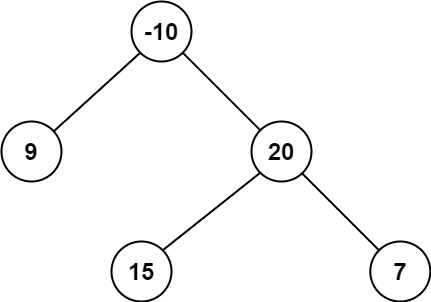# Leetcode 124 - Binary tree maximum path sum

Note:

• We def need to use DFS, but what should we return in each recursion?
• Consider these 3 situations:
•••• For each node, we need to have 3 info to decide whether we should only pick the node or extend to its subtrees.
• Base case: return [0, 0, 0].
• Normally, return max = [nodeVal, nodeVal + maxLeftSubtreeSum, nodeVal + maxRightSubtreeSum];
• Don’t really need memo because there is no repetitive calculations.
• Need to set a res variable, so we can compare with it for every node during recursions.
• res = max(res, nodeVal, nodeVal + leftSum, nodeVal + rightSum, nodeVal + leftSum + rightSum).
• What I learned from this HARD DFS question?
• Always know what you are gonna return in DFS.
• Consider simple cases to build your DFS.
• Don’t care about details too much, and don’t overthink!

Question:

A path in a binary tree is a sequence of nodes where each pair of adjacent nodes in the sequence has an edge connecting them. A node can only appear in the sequence at most once. Note that the path does not need to pass through the root.

The path sum of a path is the sum of the node’s values in the path.

Given the root of a binary tree, return the maximum path sum of any non-empty path.

Example:Code: